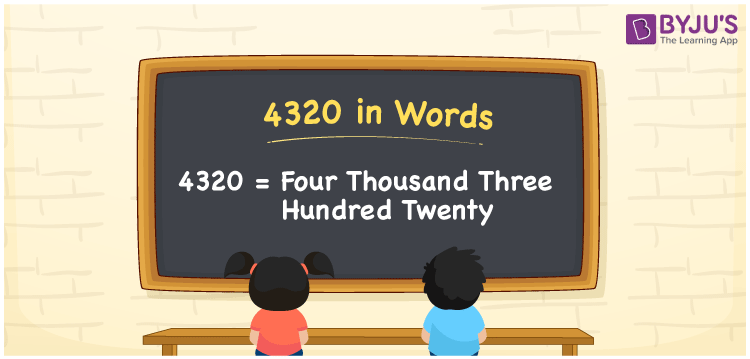# 4320 in Words

The number 4320 in words is four thousand three hundred twenty. As the number 4320 is used to represent the quantity or express the value of some object, we can say that 4320 is a cardinal number. This article will help you understand the procedure of writing the number 4320 in words using the place value system in detail.

 4320 in Words: Four Thousand Three Hundred Twenty. Four Thousand Three Hundred Twenty in Numerical Form: 4320.

## 4320 in English Words## How to Write 4320 in Words?

As discussed above, we can use the place value system for writing the number names. The below table shows the place values of the number 4320.

 Thousands Hundreds Tens Ones 4 3 2 0

The expanded form of 4320 is as follows:

= 4 × Thousand + 3 × Hundred + 2 × Ten + 0 × One

= 4 × 1000 + 3 × 100 + 2 × 10

= 4000 + 300 + 20

= 4320

= four thousand three hundred twenty

Hence, 4320 in words is four thousand three hundred twenty.

4320 in words – Four thousand three hundred twenty

Is 4320 an odd number? – No

Is 4320 an even number? – Yes

Is 4320 a perfect square number? – No

Is 4320 a perfect cube number? – No

Is 4320 a prime number? – No

Is 4320 a composite number? – Yes

## Frequently Asked Questions on 4320 in Words

### How to spell 4320 in English words?

4320 in words is four thousand three hundred twenty.

### Simplify 4000 + 320, and express it in words.

Simplifying 4000 + 320, we get 4320. Hence, 4320 in words is four thousand three hundred twenty.

### Is 4320 a prime number?

No, 4320 is not a prime number.